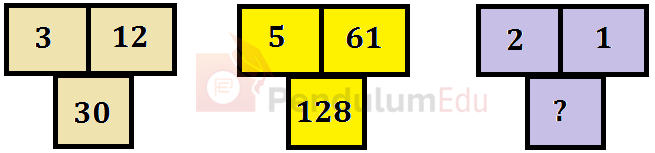Question of The Day07-10-2021

Find the missing number (?) in the third block as per the pattern followed by first two blocks.Correct Answer : d ) 14

Explanation :

Observing the number in the first block we get the pattern as,

(3)3 – 12 = 15

⇒ 15 * 2 = 30

Similarly, observing the number in the second block we get the pattern as,

(5)3 – 61 = 64

⇒ 64 * 2 = 128

Therefore, considering the third block we get,

(2)3 – 1 = 8 – 1 = 7

So,

? = 7 * 2 = 14

Hence, (d) is the correct answer.

Such type of question is asked in various government exams like SSC CGL, SSC MTS, SSC CPO, SSC CHSL, RRB JE, RRB NTPC, RRB GROUP D, UPSC CDS, UPSC NDA, UP SI etc.

Read Daily Current Affairs, Banking Awareness, Hindi Current Affairs, Word of the Day, and attempt free mock tests at PendulumEdu and boost your preparation for the actual exam.0